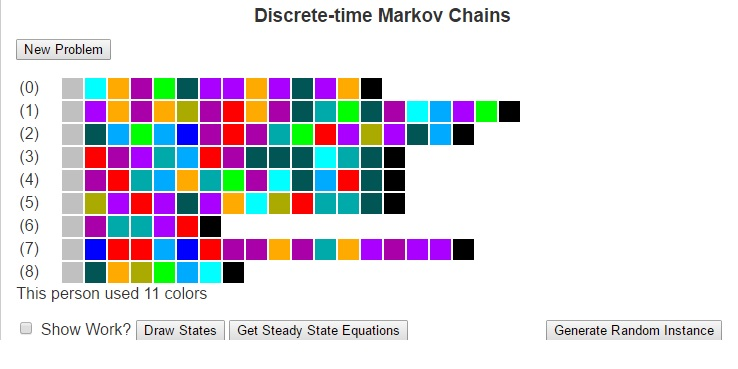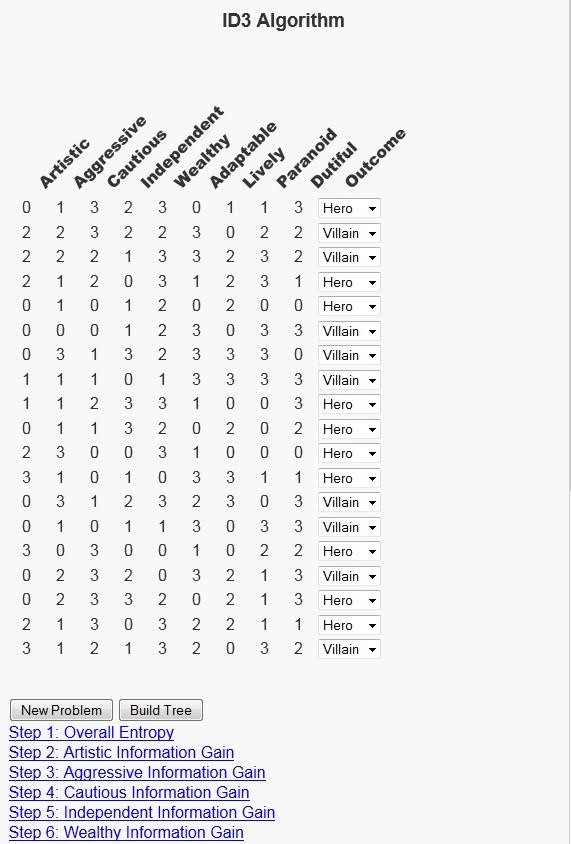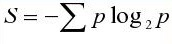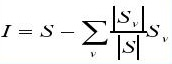# Discrete-time Markov ChainsMuch of how we interact with life could be described as transitions between states. These states could be weather conditions (whether we are in a state of “sunny” or “rainy”), the places we may visit (maybe “school”, “the mall”, “the park” and “home”), our moods (“happy”, “angry”, “sad”). There are a number of other ways to model states and even the possibility of infinitely many states.

Markov Chains are based on the principle that the future is only dependent on the immediate past. so for example, if I wished to predict tomorrow’s weather using a Markov Chain, I would need to only look at the weather for today, and can ignore all previous data. I would then compare the state of weather for today with historically how weather has changed in between states to determine the most likely next state (i.e what the weather will be like tomorrow). This greatly simplifies the construction of models.

To use Markov Chains to predict the future, we first need to compute a transition matrix which shows the probability (or frequency) that we will travel from one state to another based on how often we have done so historically. This transition matrix can be calculated by looking at each element of the history as an instance of a discrete state, counting the number of times each transition occurs and dividing each result by the number of times the origin state occurs. I’ll next give an example and then I’ll focus on explaining the Finite Discrete State Markov Chain tool I built using javascript.

Next, I want to consider an example of using Markov Chains to predict the weather for tomorrow. Suppose that we have observed the weather for the last two weeks. We could then use that data to build a model to predict tomorrow’s weather. To do this, lets first consider some states of weather. Suppose that a day can be classified in one of four different ways: {Sunny, Cloudy, Windy, Rainy}. Further, suppose that over the last two weeks we have seen the following pattern.

 Day 1 Sunny Day 2 Sunny Day 3 Cloudy Day 4 Rain Day 5 Sunny Day 6 Windy Day 7 Rain Day 8 Windy Day 9 Rain Day 10 Cloudy Day 11 Windy Day 12 Windy Day 13 Windy Day 14 Cloudy

We can look at this data and calculate the probability that we will transition from each state to each other state, which we see below:

 Rain Cloudy Windy Sunny Rain 0 1/3 1/3 1/3 Cloudy 1/2 0 1/2 0 Windy 2/5 1/5 2/5 0 Sunny 0 1/3 1/3 1/3

Given that the weather for today is cloudy, we can look at the transition matrix and see that historically the days that followed a cloudy day have been Rainy and Windy days each with probability of 1/5. We can see this more mathematically by multiplying the current state vector (cloudy) [0, 1, 0, 0] by the above matrix, where we obtain the result [1/2, 0, 1/2, 0].

In similar fashion, we could use this transition matrix (lets call it T) to predict the weather a number of days in the future by looking at Tn. For example, if we wanted to predict the weather two days in the future, we could begin with the state vector [1/2, 0, 1/2, 0] and multiply it by the matrix T to obtain [1/5, 4/15, 11/30, 1/6].

We can also obtain this by looknig at the original state vector [0, 1, 0, 0] and multiplying it by T2.

T2 =

1

 3/10 8/45 37/90 1/9 1/5 4/15 11/30 1/6 13/50 16/75 59/150 2/15 3/10 8/45 37/90 1/9

When we multiply the original state vector by T2 we arrive at this same answer [1/5, 4/15, 11/30, 1/6]. This matrix T2 has an important property in that every state can reach every other state.

In general, if we have a transition matrix where for every cell in row i and column j, there is some power of the transition matrix such that the cell (i, j) in that matrx is nonzero, then we say that every state is reachable from every other state and we call the Markov Chain regular.

Regular Markov Chains are important because they converge to what’s called a steady state. These are state vectrs x = [x0, …, xn] such that xTn = x for very large values of n. The steady state tells us how the Markov Chain will perform over long periods of time. We can use algebra and systems of linear equations to solve for this steady state vector.

For the Javascript program I’ve written, I have generated a set of painting samples for a fictional artist. The states are the different colors and the transitions are the colors that the artist will use after other colors. as well as the starting and ending colors. Given this input, we can form a Markov Chain to understand the artist’s behavior. This Markov Chain can then be used to solve for the steady state vector or to generate random paintings according to the artist’s profile. Be sure to check it out and let me know what you think.

# ID3 Algorithm Decision TreesAs I grow LEARNINGlover.com, I’m always thinking of different ways to expose my own personality through the site. This is partially because it is easier for me to talk about subjects where I am already knowledgeable, but it is more-so being done to help make some of these algorithms and concepts I encode more understandable, and sometimes relating foreign concepts to everyday life makes them easier to understand.

Today, I’d like to write about decision trees, and the ID3 algorithm for generating decision trees in particular. This is a machine learning algorithm that builds a model from a training data set consisting of a feature vector and an outcome. Because our data set consists of an outcome element, this falls into the category of supervised machine learning.

The model that the ID3 algorithm builds is called a decision tree. It builds a tree based on the features, or columns of the data set with a possible decision corresponding to each value that the feature can have. The algorithm selects the next feature by asking “which feature tells me the most about our data set?” This question can be answered first by asking how much “information” is in the data set, and then comparing that result with the amount of information in each individual feature.

In order to execute this algorithm we need a way to measure both the amount the information in outcomes of the overall data set as well as how much each feature tells us about the data set. For the first, we will use entropy, which comes from the field of information theory and encoding. Entropy is based on the question of how many bits are necessary to encode the information in a set. The more information, the higher the entropy, and the more bits required to encode that information. Although we are not encoding, the correlation between high information and high entropy suits our purposes.To understand how much each feature tells us about the outcomes of the data set we will build on the concept of entropy to define the information gain of a feature. Each feature has multiple options, so the dataset can be partitioned based on each possible value of this feature. Once we have this partition, we can calculate the entropy of each subset of the rows of data. We define the information gain of a feature as the sum over all possible outcomes of that feature can have of the entropy of that outcome multiplied by the probability of that outcome.To illustrate this algorithm, I decided to relate it to the question of whether we think of a character in a novel as a hero or villain. This is interesting because I try to read at least one book a month and as I’m reading, I often find myself asking this question about characters based on the traits of the characters as well as characters I’ve read about. In order to build an interactive script for this problem, I considered 25 possible character traits that could be present. A subset of these 25 character traits will be selected and a row will be generated grading a fictional character on a scale of 0 to 3 (0 meaning that they do not possess the trait at all, 3 meaning that the trait is very strong in their personality), and users will be asked whether they think a character with the given character traits should be listed as a hero or a villain. Then there is a button at the bottom of the script with the text “Build Tree” that executes the ID3 Algorithm and shows a decision tree that could be used to reach the set of decisions given by the user.

The possible features are:
Abstract, Adaptable, Aggressive, Ambition, Anxiety, Artistic, Cautious, Decisive, Honesty, Dutiful, Fitness, Intellect, Independent, Introverted, Lively, Open-minded, Orderly, Paranoid, Perfectionist, Romantic, Sensitive, Stable, Tension, Warmth and Wealthy

Once users select the option to build the tree, there will be several links outlining each step in the process to build this tree. These links will allow for users to expand the information relating to that step and minimize that information when done. Hopefully this will help users to understand each step more. I must say that as much fun as it has been writing this program, there were several questions when trying to explain it to others. Hopefully users get as much fun from using this tool as I had in creating it. As always feel free to contact me with any comments and or questions.

Ok, so here’s a link to the ID3 Algorithm Page. Please check it out and let me know what you think.

# Arithmetic SequencesAt a previous job of mine, there was a policy of holding a dinner party for the company each time we hired a new employee. At these dinners, each employee was treated to a \$20 dinner at the expense of the company. There was also a manager responsible for keeping track of the costs of these dinners.

In computing the costs, the manager noticed that each time there is a new dinner, it was \$20 more expensive than the last one. So if we let a1 represent the cost of the first dinner, and let ai represent the cost of the ith dinner, then we see that ai = ai-1 + 20. Sequences like this, where t arise quite often in practice and are called arithmetic sequences. An arithmetic sequence is a list of numbers where the difference between any two consecutive numbers is constant.

For the example above, the term an will represent the cost of dinner after the nth employee has joined the company (assuming that no employees have left the company over this time period). Also the term Sn will represent the total cost the company has paid towards these dinners.

Before we continue with this example, consider the following table which lists the first five terms of an arithmetic sequence as well as the common difference and the first five sums of this sequence.

 term number term value diff sum number sum value a1 4 3 S1 4 a2 7 3 S2 11 a3 10 3 S3 21 a4 13 3 S4 34 a5 16 3 S5 50

One of the beauties of arithmetic sequences is that if we know the first term (a1) and the common difference (d), then we can easily calculate the terms an and Sn for any n with the following formulas:

an = a1 + d*(n – 1), where d is the common difference.
Sn = n*(a1 + an)/2

We can use these formulas to derive more information about the sequence. For example, if my manager wanted to estimate the cost of dinners once we had added 30 new employees, this would be term a30 of the sequence, which we can evaluate with the above formula by a30 = a1 + d*(n – 1) = 0 + 20*(30 – 1) = 0 + 20 * 29 = 580.

The script is available at http://www.learninglover.com/examples.php?id=33.

Other Blogs that have covered this topic:
Study Math Online

# Learning the Apriori AlgorithmI have finished a script that runs the Apriori algorithm.

When we are given a large set of transactions, we are often interested in discovering patterns inside these transactions. The Apriori algorithm provides a means for formulating what are known as “association rules” for the set of transactions. An association rule is an observation from the database between different items inside a transaction. For example, the statement “If a customer buys chips they are 60% more likely to also purchase dip” could be an association rule based on data from supermarket purchases. The number 60% is called the confidence we have in the rule and we are generally interested in rules with higher confidence.

The Apriori algorithm takes as input a transaction database and a “threshold”. The initial pass through the database performs a count on each single item in the database and checks how many transactions contain each item. The algorithm proceeds by the Apriori property which states that “any subset of a frequent itemset must also be frequent”. What this means is that when checking the subsets of length 2 (and greater), we can ignore those subsets that contain an element that does not meet the minimum support, as it cannot be a part of a frequent itemset.

So lets look at an example. Suppose our list of transactions for the items Chips, Dip, Soda, Napkins and Paper Plates are as follows:

(Chips, Dip, Soda, Napkins, Paper Plates)
1 1 0 1 0
0 0 0 1 0
1 1 1 0 1
0 1 1 0 1
1 0 0 1 0
1 0 0 0 1
1 1 1 0 1
0 0 1 0 1
1 0 0 0 0
1 0 1 1 0
0 1 1 1 0
1 1 0 0 0
1 0 1 1 0
0 0 0 1 1
1 1 1 1 0
0 0 0 0 0
0 0 0 0 0
1 1 1 0 0
0 0 0 1 1
1 1 1 0 1

And lets suppose that we’re interested in finding the collections that occur more than a quarter (25%) of the time.

What we see from simply summing the columns and dividing by the number of columns is that 60% of the people purchased chips, 45% purchased dip, 50% purchased soda, 45% purchased napkins, and 40% purchased paper plates. Since these are all above our minimum threshold, they are all possible as elements of future collections.

When looking at larger collections, we see that:
– 35% of the people purchased both chips and dip
– 35% of the people purchased both chips and soda
– 25% of the people purchased both chips and napkins
– 35% of the people purchased both dip and soda
– 25% of the people purchased both soda and paper plates
– no other size two collections are above the minimum threshold.

You’ll note that since only 20% of the people purchased chips and paper plates, it is not above the minimum threshold and so we can ignore it in future collections. From the set of size 2 itemsets, we can derive our list of size three itemsets.

In particular, we see that:
– 25% of the people purchased all three of chips, dip, and soda.
– no other size three collections are above the minimum threshold.

The hierarchy of the itemsets is displayed in the image above.

To play around with this algorithm and understand more of its properties, visit Apriori algorithm.

Other Blogs that have covered this topic:
Analytics and Visualization of Big Data
Statistical Research

# Learn About Binary Search Trees

These data structures are organized such that the data lies in “nodes” and each node connects directly to up to two new nodes. These new nodes are called the children of the node, and the original node is called the parent. Because there are up to two children, we designate one child as the “left” child, and the other as the “right” child with the properties that the value stored in the left child is less than the value in the parent, which in itself is less than the value of its right child. If a parent has less than two children, then one (or both) of its children are given the value of null.

The insert and delete procedures need to make sure that they keep the elements of a binary search tree in sorted order.
To insert into a BST, we must first find the correct location where the new element will be placed. This means comparing the value of the new element to the current head of the tree, resulting in three possible outcomes.
if the head is null, then insert the new node at the current position because there is no subtree to compare it to.
if the value of the new element is less than the value at the head node, run the insert procedure on the left child of head.
if the value of the new element is greater than the value at the head node, run the insert procedure on the right child of head.

Similarly, the remove procedure for a binary search tree must first find the element to be removed. Once that element is found, there are three cases depending on the type of node we are dealing with.
if the node has no children, then simply remove the node from the tree.
if the node has only one child (either a left child or a right child), then have the parent of the node point to the child of the node (thus bypassing the node itself).
if the node has two children, then we have two options, either replace the node with the minimum value of the right subtree or the maximum value of the left subtree. The nodes that have these minimum and maximum values will have at most one child because by definition a value less than the minimum value in a right subtree would be a left child and thus would be less than the minimum value, contradicting the meaning of a minimum value. Because these nodes have at most one child, we can now use the procedures above to remove these nodes from the tree.
Because a binary search tree is different than a standard array, there are different methods for viewing the its contents. Three common such methods are preorder, inorder, and postorder traversal.
Preorder traversal visits the nodes of a binary search tree in the order (node), (left child), (right child).
Inorder traversal visits the nodes of a binary search tree in the order (left child), (node), (right child).
Postorder traversal visits the nodes of a binary search tree in the order (left child), (right child), (node).

We are also interested in the depth of a tree, which amounts to the amount of layers or levels of the tree. This can be computed by counting the longest path from the root of the tree to a leaf node (a node with no children) in the tree.

Other Blogs that have covered this topic:
Stoimen’s Web Log